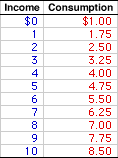Monday  June 5, 2023
 AmosWEB means Economics with a Touch of Whimsy!LONG-RUN MARGINAL COST CURVE: A graphical representation of the relationship between long-run marginal cost and the quantity of output produced. Like other marginal curves, the long-run marginal cost curve follows the average-marginal rule relative to the long-run average cost curve. In all outward appearance, the long-run marginal cost curve looks very much like the short-run marginal cost, that is, it is U-shaped. However, the U-shape is attributable to returns to scale rather than increasing and decreasing marginal returns.DERIVATION, CONSUMPTION LINE:

A consumption line, a graphical depiction of the relation between household sector consumption and income, can be derived from a simple consumption schedule, a table or chart showing the relation between household sector consumption and income. This is easily accomplished by plotting the consumption-income pairs from the schedule as points in a diagram that measures consumption on the vertical axis and income on the horizontal axis, then connection the points with a line. The consumption line can also be derived directly by plotting the consumption function using slope and intercept values.
The consumption line, also termed propensity-to-consume line or consumption function, can be derived by plotting the consumption-income combinations from a consumption schedule into a graph. It can also be derived by plotting the mathematical specification of a consumption function using slope and intercept.

Consumption ScheduleConsumption LineFirst up is the consumption schedule. A representative consumption schedule is presented in the upper panel of the exhibit to the right. The two columns in the table measure income and consumption in trillions of dollars.

The first column of this table presents total income of the household sector. These values conveniently range from \$0 to \$10 trillion. The second column then presents the amount of this income the household sector uses for consumption expenditures. These values range from \$1 trillion to \$8.5 trillion.

The derivation of the consumption line involves plotting the consumption-income pairs in the schedule into a diagram, such as the space presented in the bottom panel of the exhibit.

This currently blank space measures consumption on the vertical axis and income on the horizontal axis. The consumption-income pairs from the top panel can be plotted in the bottom panel by clicking the [Consumption Line] button.

Once all points are plotted, the result is the red consumption line labeled C. The two features of the consumption line are slope and intercept.

• Slope: The slope is positive, but less than one. In fact, the slope of the consumption line is numerically equal to the marginal propensity to consume (MPC). In this case the slope is equal to 0.75. The positive slope reflects induced consumption expenditures--more income means more consumption. Click the [Slope] button to illustrate.

• Intercept: The consumption line intersects the vertical axis at a value of \$1 trillion. This intersection point is autonomous consumption--consumption expenditures unrelated to income. Click the [Intercept] button to illustrate.
The consumption line can also be plotted directly using the consumption function. The two key bits of information needed for this task are the intercept and the slope. The following linear consumption function can be used to illustrate.
 C = a + bY
where: C is consumption expenditures, Y is income (national or disposable), a is the intercept, and b is the slope.

The consumption line can be constructed by simply drawing a line with slope b that emerges for the vertical axis at intercept a.

 <= DERIVATION, AGGREGATE EXPENDITURES LINE DERIVATION, PRODUCTION POSSIBILITIES CURVE =>Recommended Citation:

DERIVATION, CONSUMPTION LINE, AmosWEB Encyclonomic WEB*pedia, http://www.AmosWEB.com, AmosWEB LLC, 2000-2023. [Accessed: June 5, 2023].

Check Out These Related Terms...

Or For A Little Background...

And For Further Study...
Search Again?PINK FADFLY[What's This?] Today, you are likely to spend a great deal of time calling an endless list of 800 numbers trying to buy either a blue mechanical pencil or super soft, super cuddly, stuffed animals. Be on the lookout for spoiled cheese hiding under your bed hatching conspiracies against humanity.Your Complete ScopeTwo and a half gallons of oil are needed to produce one automobile tire."It takes generosity to discover the whole through others. If you realize you are only a violin, you can open yourself up to the world by playing your role in the concert. "-- Jacques Yves Cousteau, marine explorerIERInternational Economic ReviewA PEDestrian's Guide Xtra CreditTell us what you think about AmosWEB. Like what you see? Have suggestions for improvements? Let us know. Click the User Feedback link.| | | | | | | | | | |
| | | |

Thanks for visiting AmosWEB